# mini-Meucci : Applying The Checklist - Step 1

"A journey of 1000 miles begins with a single step"
Old Chinese proverb by Laozi (c. 604 BC - c. 531 BC)

### Introduction

In this mini-Meucci series of posts we'll put the 10 steps of The Checklist into practice by constructing a low volatility portfolio in Python.

This toy/basic example will be a short tourist trip, highlighting key attractions that you can then explore further...

Of course, these posts should be read in conjunction with the latest slides (I'll be referring to the slides throughout the posts) available at slideshare.net: Introduction to "The Checklist", and the former Checklist available at ssrn.com.

Better yet, attend Attilio Meucci's ARPM Bootcamp on 15-20 August 2016 in NYC and really get to know the lay of the land from the master. If you do, please give this tour guide a kind mention when you sign up!

### Toy Example Setup

Since this is a quick trip, we'll keep things relatively simple by constructing the low volatility portfolio using equities only (OK maybe I'll throw in a bond ETF later to highlight a point), and use the constituents of the Dow Jones Industrial Average as the universe.

We'll be using daily data downloaded from Yahoo and assume a 1-month (21 trading days) investment horizon.

I've also created a repo on GitHub where you can download the Python code.

### Python Setup

I'm running a Python 3.4 environment on Windows 10, installed via the Anaconda3-4.0.0 64-bit distribution. I plan on using the open source Zipline package to do the backtests in the Dynamic Allocation step.

## The Checklist Summary

The Checklist is a holistic ten-step approach to risk and portfolio management that applies i) across all asset classes; ii) to Asset Management, Banking and Insurance; iii) at portfolio and at enterprise level.
Slide #3 (slide #3 at the time I wrote this)

The 10 + 1 steps for advanced risk and portfolio management are:

• Quest for Invariance
• Estimation
• Projection
• Pricing
• Aggregation
• Evaluation
• Construction
• Execution
• Dynamic Allocation
• Ex-post Performance Analysis

The goal is to manage risk and optimize performance of a portfolio between the current time and a future investment horizon.
Slide #4

## Step 1. Quest for Invariance

The basic idea in this first step is to transform the past time series of the price data into some variables which have the nice property of IID-ness (independent and identically distributed) or invariance. In reality, we can never be sure that they are IID - I guess that's why it's called a quest...

This step has 2 parts and as it turns out, for stocks we can get a good first approximation easily.

### Step 1a. Determine the Risk Drivers

As per slide #5, take the log of the daily stock price to get its risk driver.

### Step 1b. Identify the Invariants

Slide #6: take the difference between consecutive risk driver values = change or increment = log return = continuously compounded daily return = invariant (approximately).

### Python Code Example

In :
%matplotlib inline
import numpy as np
import datetime
import matplotlib.pyplot as plt
import seaborn

# Get Yahoo data on 30 DJIA stocks and a few ETFs
tickers = ['MMM','AXP','AAPL','BA','CAT','CVX','CSCO','KO','DD','XOM','GE','GS',
'HD','INTC','IBM','JNJ','JPM','MCD','MRK','MSFT','NKE','PFE','PG',
'TRV','UNH','UTX','VZ','V','WMT','DIS','SPY','DIA','TLT','SHY']
start = datetime.datetime(2005, 12, 31)
end = datetime.datetime(2016, 5, 30)
rawdata = data.DataReader(tickers, 'yahoo', start, end)
risk_drivers = np.log(prices)
invariants = risk_drivers.diff().drop(risk_drivers.index)

# Plots
plt.figure()
prices['AAPL'].plot(figsize=(10, 8), title='AAPL Daily Stock Price (Value)')
plt.show()
plt.figure()
risk_drivers['AAPL'].plot(figsize=(10, 8),
title='AAPL Daily Log of Stock Price (Log Value = Risk Driver)')
plt.show()
plt.figure()
invariants['AAPL'].plot(figsize=(10, 8),
title='AAPL Continuously Compounded Daily Returns (Log Return = Invariant)')
plt.show()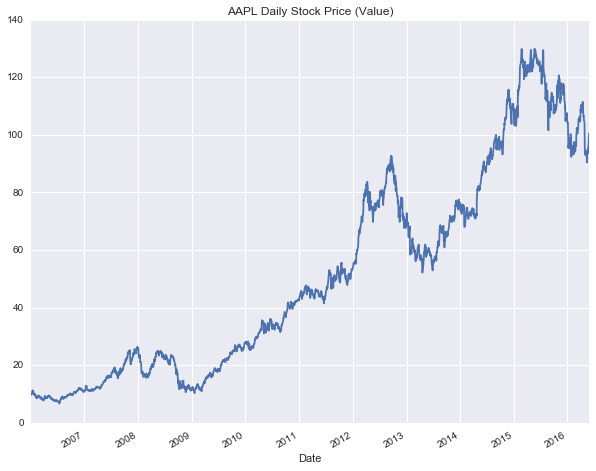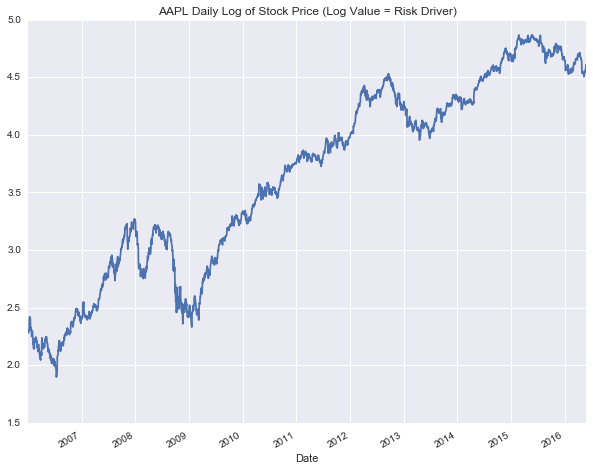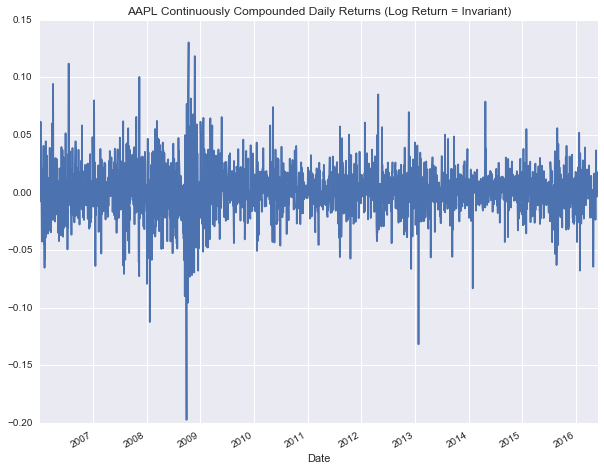# Test for Invariance

Attilio also provides a visual test for invariance. The 'bad' news is that all his code is written in Matlab.

The good news is that all his code will be written in Python going forward and made available to Bootcamp attendees... In the meantime, you're stuck with me porting (hopefully not poorly) some of it over to Python.

### Test on Simulated Data

The charts below show a test for invariance using simulated data which by construction is IID. The top 2 charts are a test for 'identically distributed' - the data is simply split in 2 halves, and if it is identically distributed the 2 histograms should look similar.

The bottom chart is testing for 'independence' or no autocorrelation (first lag) in the data. If the data is independent then the location-dispersion ellipsoid should be circular in shape (not oblong or cigar-shaped).

In :
# Test for invariance using simulated data
import rnr_meucci_functions as rnr
np.random.seed(3)
Data = np.random.randn(1000)
rnr.IIDAnalysis(Data)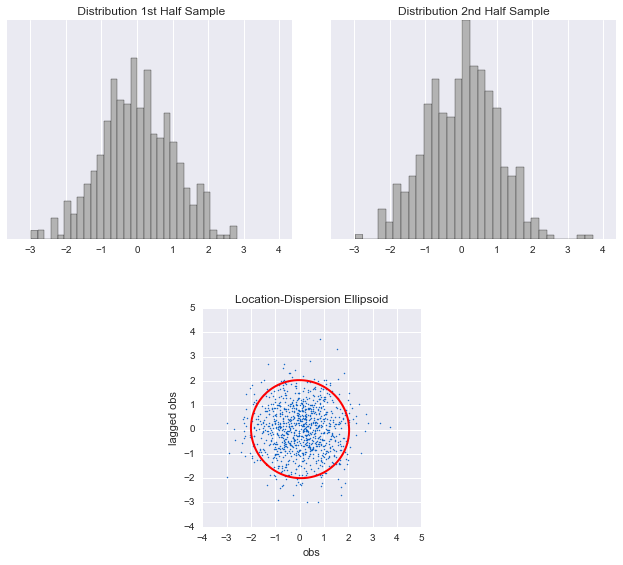### Test on Real Data

Using AAPL as an example, let's perform the tests...

In :
# Test for invariance using real data
rnr.IIDAnalysis(invariants.ix[:,'AAPL'])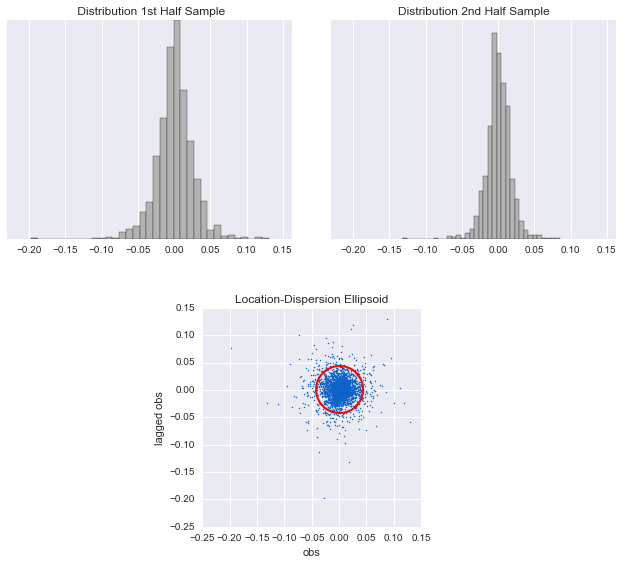And yes looks alright (approximately invariant), so we can check the first box of The Checklist. BTW, you should do this test for all the tickers - which I'll leave as an exercise for the interested readers.

Next post will be on Step 2. Estimation...

PS If you do sign-up for the Bootcamp, please let the good folks at ARPM know you learnt about it at returnandrisk.com!